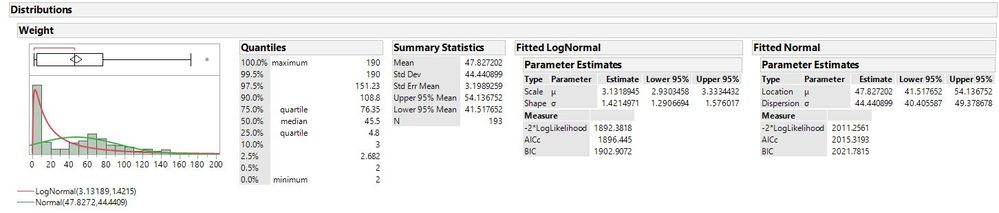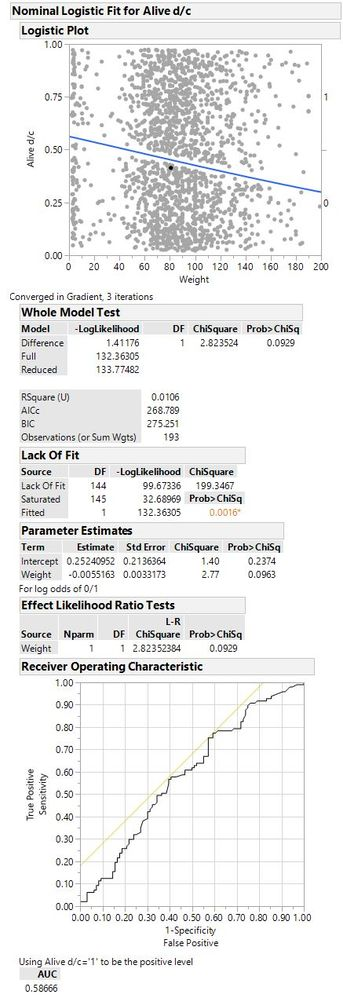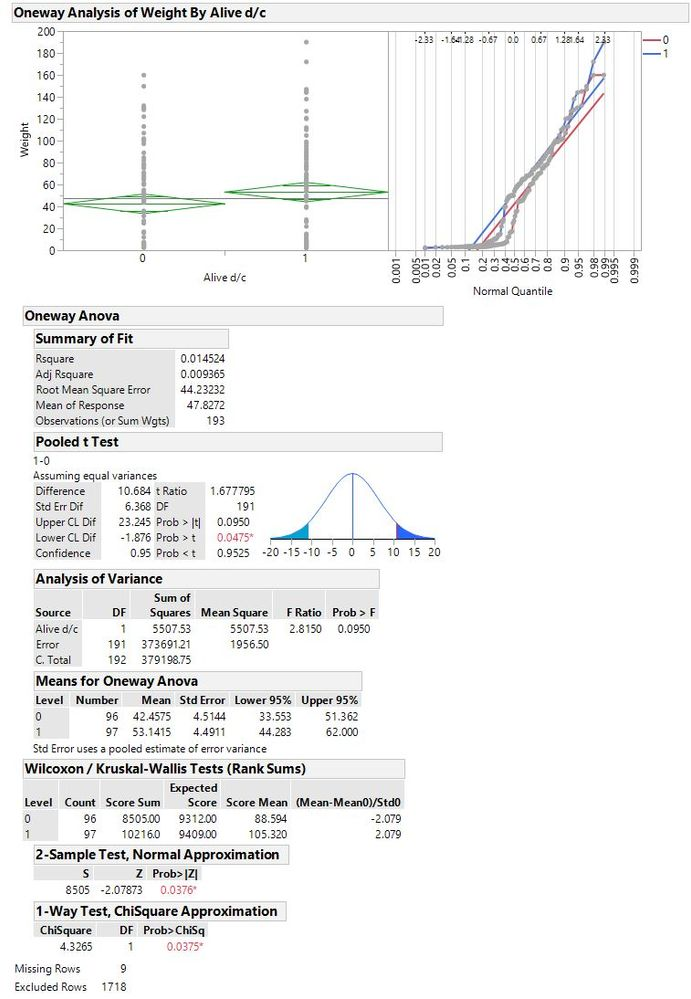Choose Language Hide Translation Bar
Highlighted

## General stat question - Nominal logistic regression vs Wilcoxon

Hello,

please help me to understand this - working with large datasets with continuous variables - most of the times I use models to a binary outcome (alive/dead).

Many of my continuous variables are not normally distributed, hence I usually approach the test by Fit Model (Y=outcome, model effects - weight, nominal logistic). The distribution of the primary variable - weight:The outcome of the logistic model looks like this:Not really a nice model, and I tried different transformations, but the distribution of my variables is a lot of time non-normally or logarithmic.

So, for better understanding for the audience, I turn the reponse and variables around: Fit Y by X (Y: Weight, X: outcome) and doing non-parametric Wilcoxon test - giving me significant result (p<0.05), while my logistic regression with a bad fit test shows a trend but no significance (p=0.09).So what do ?

The response is the clearly the outcome (Y), so should I stick to this test even when the lack of fit test is positive. Many readers are not used to logistic regression and rather prefer quantile charts , but this would require the outcome to be weight (Y).

Which test would be legit to use ?

Can I overcome the lack of fit in the logistic regression ?

Which p value can I use at the end ? - is there a way to define OR for non-parametric test results ?

Thanks a lot, Marc

3 REPLIES 3
Highlighted

## Re: General stat question - Nominal logistic regression vs Wilcoxon

First, I would say: forget all about the different statistical techniques and how you might use them. I have a very fundamental question: What is the purpose of your analysis? What are you trying to do? What would you like to know? Starting with those questions will then guide the proper analysis and what (if anything) you need to do with non-normal variables. Note that in many cases, normality is not really required.

Dan Obermiller
Highlighted

## Re: General stat question - Nominal logistic regression vs Wilcoxon

The question is always if certain predictors are linked to a certain outcome (most in my field - death / alive). In such retrospective data analysis, we usually have many potential demographic, clinical variables , which are linked to a certain outcome (death / alive). Weight in this example was one of the variables as an example.

Highlighted

## Re: General stat question - Nominal logistic regression vs Wilcoxon

"Linked" could mean many things. You could just look at a correlation value and say the correlation is high, so variables are linked. I think you are really looking to see what CAUSES your outcome. That implies a modeling approach like you started with. Outcome is a Y. Everything else is an X. I would recommend looking at all possible inputs simultaneously to avoid some relationships not appearing due to correlations among the inputs or some relationships appearing, but being caused by a lurking variable.

As for normality, that is NOT a requirement for your input variables. Transforming inputs that are highly skewed may help the modeling though, in some cases. The example that you show, I would not be that concerned with the weight, but there is a very large spike at the beginning (near 2) that may be problematic. You could try taking the log of weight and use that as your input to see if it changes your results.

You could also try Discriminant Analysis (DA), which is similar to logistic regression. It answers the question, can I find a multivariate hyperplane that best separates the data into the two outcome values. It would be the proper technique rather than the Oneway ANOVA and handles multiple possible factors simultaneously. DA can be more powerful than logistic regression, but relies more heavily on data being normally distributed. It may be another tool for you to try, but due to the non-normality, I would try transforming first before DA.

Dan Obermiller
Article Labels

There are no labels assigned to this post.